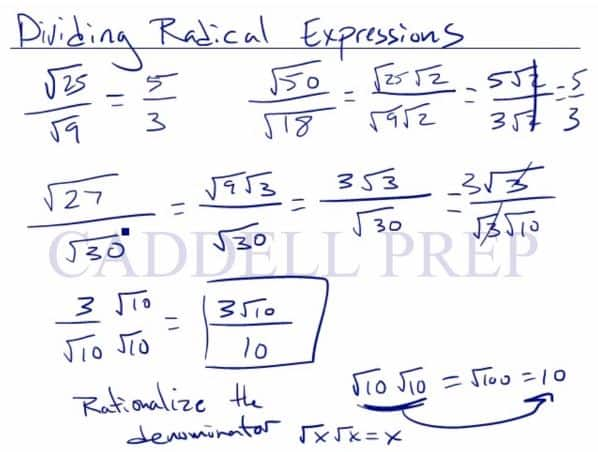In this video, we are going to divide radical expressions. After you finish this lesson, view all of our Algebra 1 lessons and practice problems.

One simple example is:$\dfrac{\sqrt{25}}{\sqrt{9}}$
By simplifying the numerator and the denominator, we now have$\dfrac{5}{3}$

A slightly more difficult problem would be:$\dfrac{\sqrt{50}}{\sqrt{18}}$
Just like the other problem, first find perfect square factors for each expression$\dfrac{\sqrt{25}\times\sqrt{2}}{\sqrt{9}\times\sqrt{2}}$
Simplify each expression$\dfrac{5\sqrt{2}}{3\sqrt{2}}$
And the final answer is$\dfrac{5}{3}$

If the expression has a radical in the denominator and a rational number in the numerator, then it is necessary to rationalize the fraction.
For example:$\dfrac{3}{\sqrt{10}}$
Multiple the denominator to both the numerator and the denominator. Like terms cancel each other out so the final answer is:$\dfrac{3\times\sqrt{10}}{\sqrt{10}\times\sqrt{10}}$$\dfrac{3\sqrt{10}}{10}$Example 1$\dfrac{3}{8\sqrt{2}}$
Since the denominator has a radical, we have to rationalize the fraction.
Multiply the numerator and denominator by$\sqrt{2}$$\dfrac{3}{8\sqrt{2}}\cdot\dfrac{\sqrt{2}}{\sqrt{2}}$
Now, we have$\dfrac{3\sqrt{2}}{8(2)}$$\dfrac{3\sqrt{2}}{16}$

Example 2$\dfrac{3xy^2}{\sqrt{25x^3y}}$
Simplify first the terms inside the radical$\dfrac{3y}{\sqrt{25x^2}}$
Then$\sqrt{25}$ is$5$ and$\sqrt{x^2}$ is$x$
Now, we have$\dfrac{\sqrt{3y}}{5x}$

Video-Lesson Transcript

Let’s go over how to divide radical expressions.

Let’s say we have$\dfrac{\sqrt{25}}{\sqrt{9}}$$\dfrac{5}{3}$

And there’s not much to do.

But what if we have$\dfrac{\sqrt{50}}{\sqrt{18}}$$= \dfrac{\sqrt{25} \sqrt{2}}{\sqrt{9} \sqrt {2}}$$= \dfrac{5 \sqrt{2}}{3 \sqrt{2}}$

And just like if we have$\dfrac{5x}{3x}$$x$ will be cancelled out.

In this case,$\sqrt{2}$ will be cancelled out.$= \dfrac{5}{3}$

Of course, not all is going to work out like this.

Let’s look at another example.$\dfrac{\sqrt{27}}{\sqrt{30}}$

Now, let’s break this down.$= \dfrac{\sqrt{9} \sqrt{3}}{\sqrt{30}}$$= \dfrac{3 \sqrt{3}}{\sqrt{30}}$

This is how far we can go as far as the radicals are concerned.

But this is not our final answer.

Because we don’t want a radical in the denominator.

But even more so, we can reduce this.

Just like this example:$\dfrac{\sqrt{15}}{\sqrt{5}}$$= \dfrac{\sqrt{3}}{\sqrt{1}}$$= \sqrt{3}$

Another way to look at that is:$\dfrac{\sqrt{15}}{\sqrt{5}}$$= \dfrac{\sqrt{5} \sqrt{3}}{\sqrt{5}}$$\sqrt{5}$ cancels out and we’re left with$= \sqrt{3}$

Now, let’s apply this in solving. Let’s go back$= \dfrac{3 \sqrt{3}}{\sqrt{30}}$

We can break this down even more into$= \dfrac{3 \sqrt{3}}{\sqrt{3} \sqrt{10}}$

Here,$= \sqrt{3}$ is going to cancel out.

And we have$= \dfrac{3}{\sqrt{10}}$

Now, we want to rationalize the denominator.

The trick in getting rid of$\sqrt{10}$ is to multiply the numerator and denominator by the same number.$= \dfrac{3 \times \sqrt{10}}{\sqrt{10} \times \sqrt{10}}$$= \dfrac{3 \sqrt{10}}{10}$

Now, let’s look at some more.$= \dfrac{ \sqrt{45}}{\sqrt{15}}$

Let’s break this down$= \dfrac{\sqrt{9} \sqrt{5}}{\sqrt{15}}$$= \dfrac{3 \sqrt{5}}{\sqrt{15}}$

Then let’s reduce$5$ and$15$

We’ll have$= \dfrac{3 \sqrt{1}}{\sqrt{3}}$$= \dfrac{3}{\sqrt{3}}$

Now, we don’t want a radical in the denominator.

We have to rationalize it. So let’s multiply the numerator and denominator by$\sqrt{3}$.$= \dfrac{3 \times \sqrt{3}}{\sqrt{3} \times \sqrt{3}}$$= \dfrac{3 \sqrt{3}}{3}$

Which we cancel and we’re left with our final answer$= \sqrt{3}$

This is a$100\%$ correct way to solve it.

Any way you solve for the correct answer is a good way to do it.

Let me show you another way.

We can reduce this right off the bat.$= \dfrac{ \sqrt{45}}{\sqrt{15}}$$15$ goes into$45$, so this can be reduced to$= \dfrac{ \sqrt{3}}{\sqrt{1}}$$\sqrt{3}$

Two different options to solve the same problem. We have the same answer both ways.